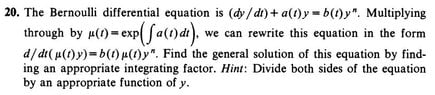# Help with a question (Bernoulli General solution)

• MHB
• Ultra
Hello, Thanks for your reply. I am currently reading a text book on partial derivatives. Any Calculus level text (you are looking for something like Calc III) should do the trick. I'm not certain that you are going to find a source on just partials but there ought to be any number of videos and examples online.

#### Ultra

Hello guys I hope you all are doing well. :)

I found below question in a book by Martin Braun "Differential Equations and Their Applications An Introduction to Applied Mathematics (Fourth Edition)"

The question :
The Bernoulli differential equation is (dy/dt)+a(t)y=b(t)y^n. Multiplying through by µ(t)=exp(Integral of a(t).dt), we can rewrite this equation in the form
d/dt(µ(t)y)=b(t)µ(t)y^n. Find the general solution of this equation by finding an appropriate integrating factor. Hint: Divide both sides of the equation
by an appropriate function of y.

I have problems solving this question. because this question is located in section (1.9) of the book which talks about exact equations and my problem is that
I cannot turn "d/dt(µ(t)y)=b(t)µ(t)y^n" to an exact equation form. I'd appreciate any help. :)

#### Attachments

•Question_20 (section 1.9) .jpg
28.9 KB · Views: 87
Welcome, Ultra!

Multiply both sides of the Bernoulli equation by $y^{-n}$ to obtain $y^{-n} dy/dt + a(t)y^{1-n} = b(t)$. By the chain rule, $d/dt(y^{1-n}) = (1-n)y^{-n} dy/dt$. So the equation can be written $d/dt(y^{1-n}) + (1-n)a(t)y^{1-n} = (1-n) b(t)$. If $u = y^{1-n}$, then $du/dt + (1-n)a(t)u = (1-n)b(t)$. In this form, you can now find an appropriate integrating factor and solve the equation.

Hello Euge,

#### Attachments

• Question_20_section_1.9.pdf
150.3 KB · Views: 86
If $z = \mu y$, then $y = z/\mu$ and $d/dt(\mu(t)y) = b(t)\mu(t)y^n$ becomes $$\frac{dz}{dt} = \frac{b}{\mu^{n-1}} z^n$$ Alternatively, $$\frac{dz}{z^n} - \frac{b(t)}{\mu(t)^{n-1}}\, dt = 0$$ This equation is already exact, in fact, separable. So there is no integrating factor to be found.

Hello Euge,

And thank you for your reply. So, the attached PDF is totally wrong?

#### Attachments

• Question_20_section_1.9_2.pdf
143.1 KB · Views: 95
Ultra said:
Hello Euge,

And thank you for your reply. So, the attached PDF is totally wrong?
Everything up to the point where you say "And" is correct. But pretty much everything below the statement "Now could we say below" is not. When you take your partial with respect to t you will not get the RHS. I'm not sure how you calculated it, but it's wrong.

-Dan

topsquark said:
Everything up to the point where you say "And" is correct. But pretty much everything below the statement "Now could we say below" is not. When you take your partial with respect to t you will not get the RHS. I'm not sure how you calculated it, but it's wrong.

-Dan
Hello Dan,

And thank you.

topsquark said:
Everything up to the point where you say "And" is correct. But pretty much everything below the statement "Now could we say below" is not. When you take your partial with respect to t you will not get the RHS. I'm not sure how you calculated it, but it's wrong.

-Dan
Dan what book u suggest for someone who wants bone up his understanding of partials? (after all these years I still think I haven't known them by heart!)

Ultra said:
Dan what book u suggest for someone who wants bone up his understanding of partials? (after all these years I still think I haven't known them by heart!)
Any Calculus level text (you are looking for something like Calc III) should do the trick. I'm not certain that you are going to find a source on just partials but there ought to be any number of videos and examples online.

-Dan